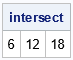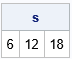To get better at something, you need to practice. That maxim applies to sports, music, and programming. If you want to be a better programmer, you need to write many programs. This article provides an example of forming the intersection of items in a SAS/IML list. It then provides several exercises so that SAS/IML programmers can practice working with lists.

### What are SAS/IML lists?

A SAS/IML list is a data structure that enables you to pack various SAS/IML objects (scalars, vectors, matrices, tables, ...) into a single object. A primary use of lists is to pass information between functions. You can create lists and extract items from lists by using a special syntax. For example, if L is a list then L\$1 is the first item in the list, L\$2 is the second item, and so on.

Recently, a SAS/IML programmer asked some basic questions about manipulating items in a list. The chapter about lists in the SAS/IML User's Guide includes many examples, but there is a big difference between reading documentation and writing a program yourself. This article provides exercises that enable you to practice working with lists.

### The intersection of items in a list

This section shows how to compute the intersection of items in a list. The input is a SAS/IML list that contains many matrices. The output is the intersection between the items.

If you have a set of matrices, the XSECT function in SAS/IML computes the intersection of the values in the matrices. However, you cannot pass a list to the XSECT function. The following example shows that the XSECT function takes a comma-separated set of matrices:

```proc iml; x = 1:20; /* 1,2,3,...,20 */ y = do(0, 20, 2); /* 0,2,4,...,20 */ z = do(0, 21, 3); /* 0,3,6,...,21 */ w = 3:24; /* 3,4,5,...,24 */   intersect = xsect(x,y,z,w); print intersect;```The intersection of the matrices (thought of as sets) contains three elements. Notice that the arguments to the XSECT function are matrices.

So, how could you compute the intersection of matrices if they are items in a list? The key is to recognize that the intersection of k sets is equal to taking k-1 intersections. First, form S1 as the intersection of X and Y. Then form S2 as the intersection of S1 and Z. Lastly, form S3 (the answer) as the intersection of S2 and W. Thus, you can compute the total intersection by always computing the intersection of pairs of sets.

Thus, you can compute the intersection by looping over the items in the list. You can use the ListLen function to get the number of items in a list. Then repeatedly compute the pairwise intersections, as follows:

```start ListIntersect(L); len = ListLen(L); if len=0 then /* no items in list */ return ( {} ); /* return empty matrix */ else if len=1 then /* one item */ return L\$1; /* return the item */   intersect = xsect(L\$1, L\$2); /* the intersection of the first 2 items */ do i = 3 to len; intersect = xsect(intersect, L\$i); /* the intersection of the first i items */ end; return intersect; finish;   /* test the function: pack matrices into a list */ L = [x, y, z, w]; s = ListIntersect(L); print s;```Success! The ListIntersect function returns the same values as the original call to the XSECT function.

### Exercises for manipulating lists

1. The previous example shows that the ListIntersection function works on a list that contains numerical matrices. But the XSECT function also works for character values. Use the following character matrices to test whether the ListIntersect function works for a list that contains character items. If not, modify the function so that it works for a list of character matrices.
```a = 'A':'Z'; b = {A C E G I K M O Q S U W Y}; c = {A B C F G I K N O R S V Y}; d = {I THINK IT IS EITHER X OR Y};```
2. The ListIntersect function does not check that the items in the list are the same type. Use the TYPE function to ensure that all items in the list have the same type, either all numeric or all character. If not, return the empty matrix.
3. Write a function called ListUnion that uses the UNION function to compute the union of the elements in a list. Make sure it handles lists that contain all numeric or all character items.
4. Given a matrix (m) and a list (L), you can check to see which items in the list have a nonempty intersection with the matrix. If there are N items in the list, write a function called HasIntersection that returns a binary vector (v) of length N. The i_th value, v[i], is 1 if XSECT(m, L\$i) is nonempty. Otherwise, v[i]is 0. For example, the following call should return the vector {0 1 1 1} because L has four items and m has a nonempty intersection with items 2, 3, and 4:
```m = {0 24}; v = HasIntersection(m, L);```

### Summary

If you want to be good at something, you need to practice. This article shows how to manipulate items in a SAS/IML list to form the intersection of items. It then provides a set of exercises that a motivated programmer can use to practice working with lists.

The post Operations on lists in SAS/IML appeared first on The DO Loop.